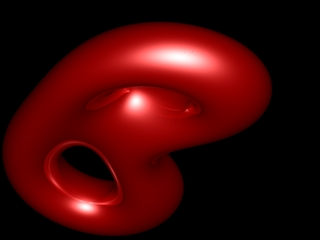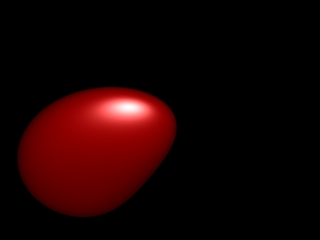# 4D torus

A 3D torus is a donut shape. The 'skin' of a torus can be made by spinning a circle around an axis outside the circle. In topology, you'd say its a circle times a circle. This is really a 2D thing. The skins of 3D things are 2D things.

A four-dimensional torus has a skin which is a circle times a circle times a circle. You can't build a model of a 4D torus in 3D space, any more than you can build a 3D shape inside a thin plane. But if you slice the 4D shape, you get a 3D slice.

To get the pictures on this page, I took lots of slices of a 4D torus. I made them into posters and movies, for you to enjoy.

Some more details :

The equation for the torus shown is

• W = (4 + (2+cos(a))cos(b))cos(c)
• X = (4 + (2+cos(a))cos(b))sin(c)
• Y = (2+cos(a))sin(b)
• Z = sin(a)
I sliced it along "planes" with equations X+Y+Z+W = p. These "planes" are really 3D spaces inside the 4D space. You can describe points in these planes with 3 coordinates x,y,z.

I converted the equations for W, X, Y, and Z to a single polynomial equation in x, y, z and p. The original system was really a system of 17 polynomial equations, in 14 variables. The variables are W, X, Y, Z, x, y, z, p, and also sin(a), cos(a), sin(b), etc. I had help from computers to reduce them. I used this polynomial reducer to eliminate variables one by one, and maxima to factorise the results each step.

Then, I put my polynomial equation into POV-Ray, to produce the nice pictures you see on this page.

Feel free to download the pictures and use them as wallpapers or screensavers, etc, etc. Or buy a poster to hang in your home or office. Or, watch the videos. They're all here for you to enjoy!

### Posters

Here's some posters available for you to enjoy

### Pictures

Here's a set of pictures of the 4d torus. Click on a picture for a larger version.